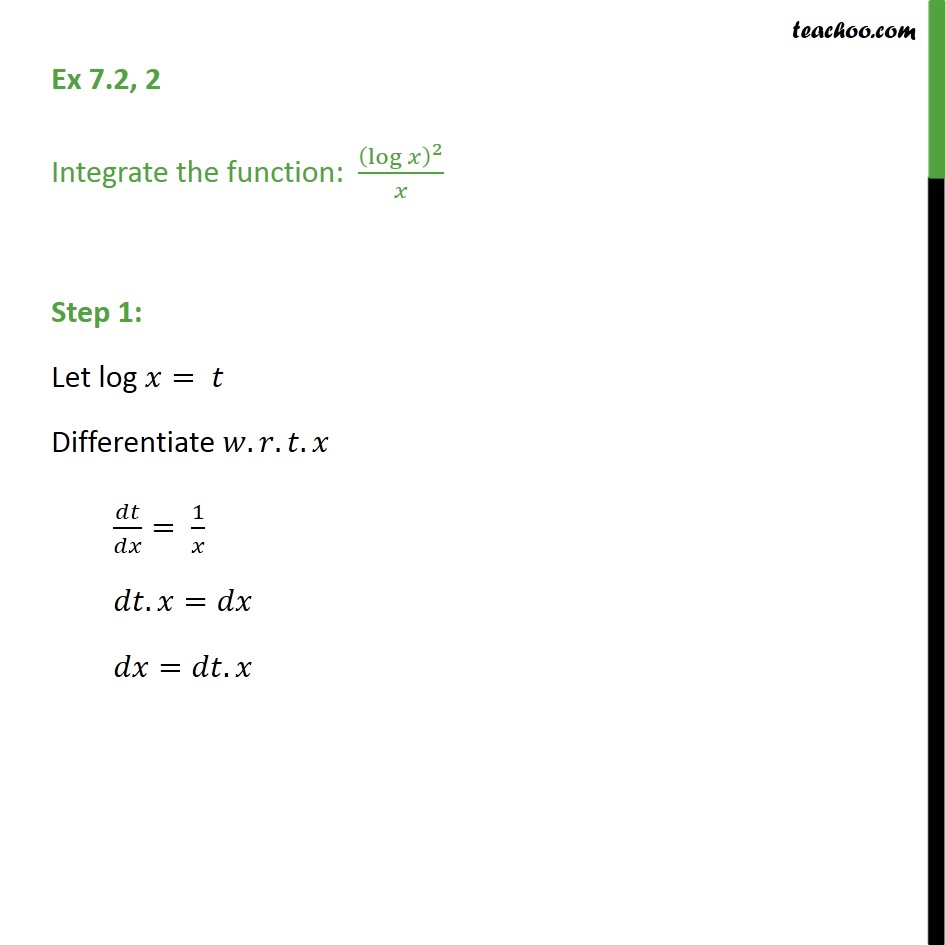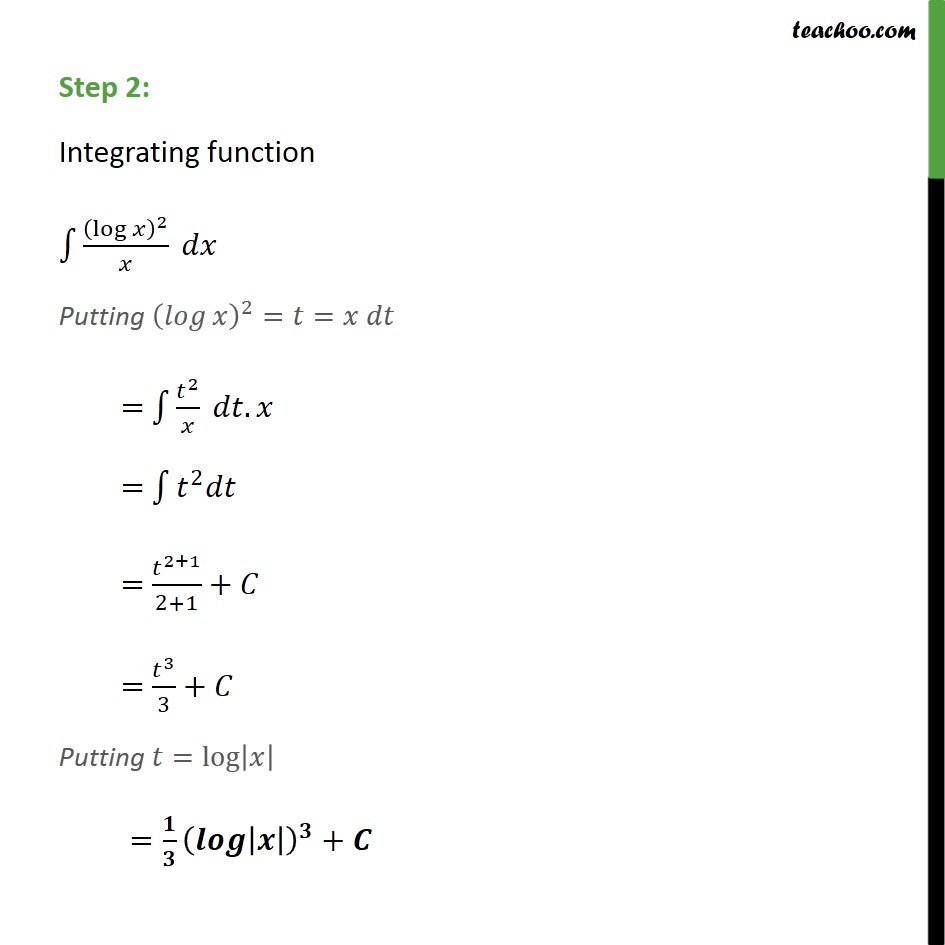1. Chapter 7 Class 12 Integrals (Term 2)
2. Serial order wise
3. Ex 7.2

Transcript

Ex 7.2, 2 Integrate the function: (log⁡𝑥 )^2/𝑥 Step 1: Let log 𝑥= 𝑡 Differentiate 𝑤.𝑟.𝑡.𝑥 𝑑𝑡/𝑑𝑥= 1/𝑥 𝑑𝑡.𝑥=𝑑𝑥 𝑑𝑥=𝑑𝑡.𝑥 Step 2: Integrating function ∫1▒(log⁡𝑥 )^2/𝑥 𝑑𝑥 Putting (𝑙𝑜𝑔⁡𝑥 )^2=𝑡=𝑥 𝑑𝑡 = ∫1▒𝑡^2/𝑥 𝑑𝑡.𝑥 = ∫1▒〖𝑡^2 𝑑𝑡〗 = 𝑡^(2+1)/(2+1)+𝐶 = 𝑡^3/3+𝐶 Putting 𝑡=log⁡|𝑥| =𝟏/𝟑 (𝒍𝒐𝒈⁡|𝒙| )^𝟑+𝑪

Ex 7.2